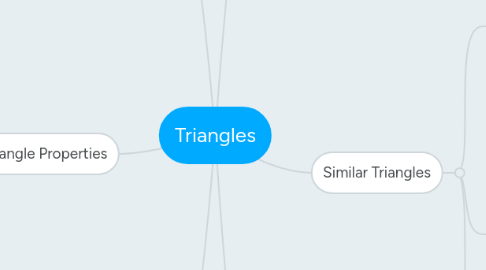# TrianglesZach Wang
Get Started. It's FreeTriangles## 1. Classifying Triangles

### 1.1. By

1.1.1. Side

1.1.1.1. Scalene

1.1.1.1.1. No congruent sides

1.1.1.2. Isosceles

1.1.1.2.1. Two congruent sides

1.1.1.3. Equilateral

1.1.1.3.1. All sides and angles congruent

1.1.2. Angle

1.1.2.1. Acute

1.1.2.1.1. All angles are acute

1.1.2.2. Obtuse

1.1.2.2.1. One angle is obtuse

1.1.2.3. Right

1.1.2.3.1. One angle is right

## 2. Triangle Properties

### 2.1. Lines in Triangles

2.1.1. Altitudes

2.1.1.1. The perpendicular segment from a vertex to an opposite side. Also known as height.

2.1.1.2. Orthcenter

2.1.1.2.1. The point of intersection of the three altitudes

2.1.2. Angle Bisector

2.1.2.1. The line that divides an angle in half

2.1.2.2. Incenter

2.1.2.2.1. The point of intersection of the three angle bisectors

2.1.3. Medians

2.1.3.1. The segment whose end points are a vertex and the midpoint of an opposite side

2.1.3.2. Centroid

2.1.3.2.1. The point of intersection of the three medians

2.1.4. Perpendicular BIsector

2.1.4.1. The line perpendicular to a side at its midpoint

2.1.4.2. Circumcenter

2.1.4.2.1. The point of intersection of the three perpendicular bisectors

### 2.2. Midsegments

2.2.1. The mid-segment of a triangle is a segment joining the midpoints of two sides of a triangle

2.2.2. Midsegment Theorem

2.2.2.1. The segment joining the midpoints of any two sides will be parallel to the third side and half its length

### 2.3. Hinge Theorem

2.3.1. If two triangles have two pairs of sides which are the same length, the triangle with the larger included angle also has the larger third side. longer than the third side of the second triangle

### 2.4. Inequalities

2.4.1. AB + BC > AC

2.4.2. AB + AC > BC

2.4.3. CB + AC > AB

## 3. Angles and Triangles

### 3.1. Theorems

3.1.1. Triangle Sum Theorem

3.1.1.1. The sum of the measures of the interior angles of a triangle is always 180 degrees

3.1.2. Triangle Exterior Angle Theorem

3.1.2.1. The measure of an exterior angle of a triangle is equal to the sum of the measures of the non-adjacent interior angles

3.1.3. Third Angle Theorem

3.1.3.1. If two angles of one triangle are congruent to two angles of another triangle, then the third triangle angles are also congruent

## 4. Congruent Triangles

### 4.1. Properties of Congruence

4.1.1. Reflexive Property of Congruence

4.1.1.1. A quantity is congruent to itself.  a = a

4.1.2. Transitive Property of Congruence

4.1.2.1. If a = b, and b = c, then a = c

4.1.3. Symmetric Property of Congruence

4.1.3.1. If a = b, then b = a.

### 4.2. Proving Triangles Congruent

4.2.1. Congruence Postualtes

4.2.1.1. Side Side Side Postulate (SSS)

4.2.1.1.1. If three sides of one triangle are congruent to three sides of another triangle, the triangles are congruent

4.2.1.2. Side Angle Side Postulate (SAS)

4.2.1.2.1. If two sides and the included angle of one triangle are congruent to the corresponding parts of another triangle, the triangles are congruent

4.2.1.3. Angle Side Angle Postulate (ASA)

4.2.1.3.1. If two angles and the included side of one triangle are congruent to the corresponding parts of another triangle, the triangles are congruent

4.2.2. Congruence Theorems

4.2.2.1. Angle Angle Side Theorem (AAS)

4.2.2.1.1. If two angles and the non-included side of one triangle are congruent to the corresponding parts of another triangle, the triangles are congruent

4.2.2.2. Hypotenuse Length Theorem (HL)

4.2.2.2.1. If the hypotenuse and leg of one right triangle are congruent to the corresponding parts of another right triangle, the right triangles are congruent

## 5. Similar Triangles

### 5.1. Proving Triangles Similar

5.1.1. Theorems

5.1.1.1. Side Side Side (SSS~)

5.1.1.1.1. If the three sets of corresponding sides of two triangles are in proportion, the triangles are similar

5.1.1.2. Side Angle Side (SAS~)

5.1.1.2.1. If an angle of one triangle is congruent to the corresponding angle of another triangle and the lengths of the sides including these angles are in proportion, the triangles are similar

5.1.1.3. Angle Angle (AA~)

5.1.1.3.1. If two angles of one triangle are congruent to two angles of another triangle, the triangles are similar

### 5.2. Solving for Missing Sides

5.2.1. Side Splitter Theorem

5.2.1.1. If a line is parallel to a side of a triangle and it intersects the two other sides, then the line divides these two sides proportionally

5.2.2. Triangle Angle Bisector Theorem

5.2.2.1. An angle bisector divides the sides of a triangle proportionally

5.2.3. To find missing side lengths in similar triangles,

5.2.4. Step 1: Find the ratio of corresponding sides in pairs of similar triangles.

5.2.5. Step 2: Use that ratio to find the unknown lengths.

### 5.3. Geometric Means

5.3.1. The geometric mean of a set of numbers is all the numbers multiplied, then the nth root of the product

5.3.2. Geometric Mean Theorem

5.3.2.1. The geometric mean of two segments equals the altitude

## 6. Right Triangles

### 6.1. Pythagorean Theorem

6.1.1. a^2 + b^2 = c^2

### 6.2. Special Triangles

6.2.1. 30-60-90 Triangles

6.2.1.1. If the short leg is 1, then the long leg is root3, and the hypotenuse is 2

6.2.2. 45-45-90 Triangles (Isosceles Right Triangle)

6.2.2.1. If the two congruent sides are 1, then the hypotenuse is root2

### 6.3. Trigonometric Ratios

6.3.1. Sine

6.3.1.1. sin(x) = o/h

6.3.2. Cosine

6.3.2.1. cos(x) = a/h

6.3.3. Tangent

6.3.3.1. tan(x) = o/a

6.3.4. Cosecant

6.3.4.1. csc(x) = h/o

6.3.5. Secant

6.3.5.1. sec(x) = h/a

6.3.6. Cotangent

6.3.6.1. cot(x) = a/o